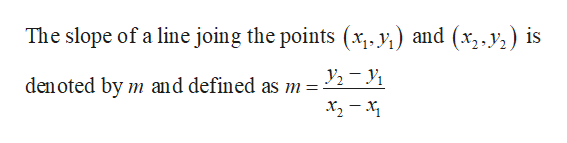# Let P= (2,3) and Q= (4,6). Find the slope of the line containing P and Q?

Question

Let P= (2,3) and Q= (4,6). Find the slope of the line containing P and Q?

check_circleExpert Solution
Step 1

Formula:...help_outlineImage TranscriptioncloseThe slope of a line joing the points (x, y) and (x,y) is den oted by m and defined as m =- fullscreen

### Want to see the full answer?

See Solution

#### Want to see this answer and more?

Solutions are written by subject experts who are available 24/7. Questions are typically answered within 1 hour*

See Solution
*Response times may vary by subject and question
Tagged in

### Other